Main Content

# Types of Bar Graphs

Bar graphs are useful for viewing results over a period of time, comparing results from different data sets, and showing how individual elements contribute to an aggregate amount.

By default, bar graphs represents each element in a vector or matrix as one bar, such that the bar height is proportional to the element value.

### 2-D Bar Graph

The `bar` function distributes bars along the x-axis. Elements in the same row of a matrix are grouped together. For example, if a matrix has five rows and three columns, then `bar` displays five groups of three bars along the x-axis. The first cluster of bars represents the elements in the first row of Y.

```Y = [5,2,1 8,7,3 9,8,6 5,5,5 4,3,2]; figure bar(Y)```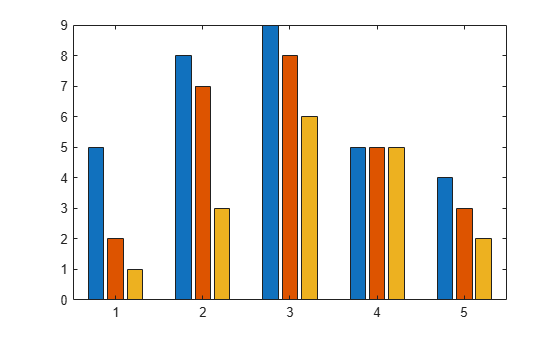To stack the elements in a row, specify the `stacked` option for the `bar` function.

```figure bar(Y,'stacked')```### 2-D Horizontal Bar Graph

The `barh` function distributes bars along the y-axis. Elements in the same row of a matrix are grouped together.

```Y = [5,2,1 8,7,3 9,8,6 5,5,5 4,3,2]; figure barh(Y)```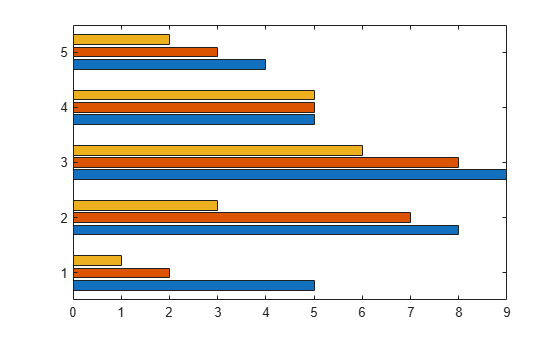### 3-D Bar Graph

The `bar3` function draws each element as a separate 3-D block and distributes the elements of each column along the y-axis.

```Y = [5,2,1 8,7,3 9,8,6 5,5,5 4,3,2]; figure bar3(Y)```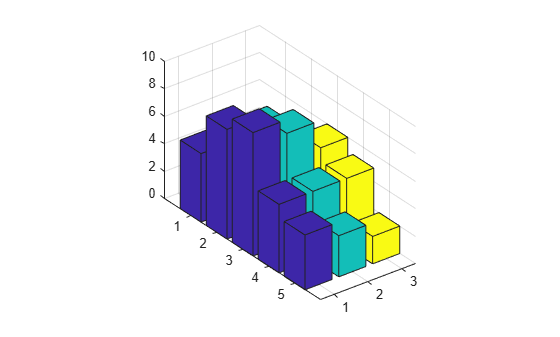To stack the elements in a row, specify the `stacked` option for the `bar3` function.

```figure bar3(Y,'stacked')```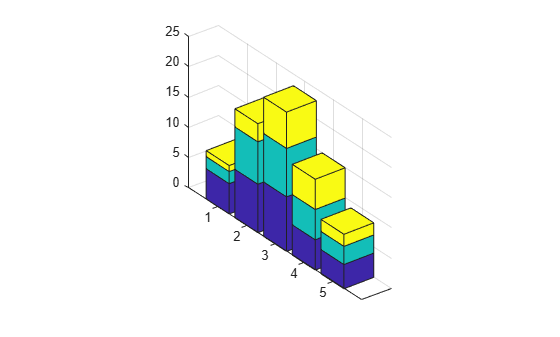### 3-D Horizontal Bar Graph

The `bar3h` function draws each element as a separate 3-D block and distributes the elements of each column along the z-axis.

```Y = [5,2,1 8,7,3 9,8,6 5,5,5 4,3,2]; figure bar3h(Y)```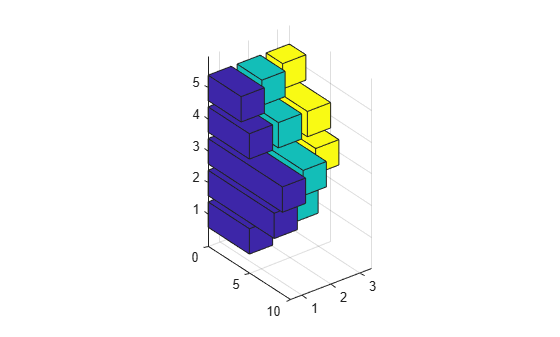Download ebook